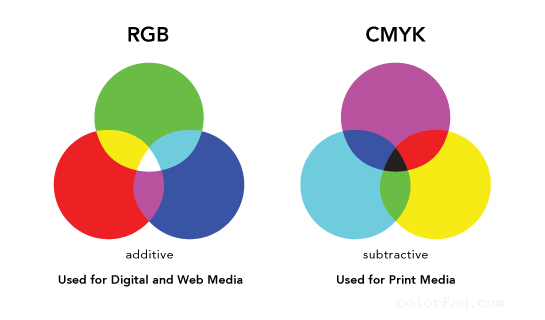The CMYK color model is a subtractive color model used in color printing. CMYK refers to the four inks used in some color printing: cyan, magenta, yellow, and key (black). In additive color models such as RGB (for example, for computer monitors), white is the "additive" combination of all primary colors, while black is the absence of light. In the CMYK model, the situation is just the opposite: inked (white) paper absorbs or reflects specific wavelengths, so cyan, magenta, and yellow pigments act as filters, subtracting different degrees of red, green, and blue from white light to produce selectivity Color gamut spectral colors (the CMYK color model is called subtractive color because the ink "subtracts" the brightness from white).### RGB to CMYK

1

<?php

2

/*

3

Convert Between RGB and CMYK Color Values with PHP

4

5

*/

6

7

function beliefmedia_rgb_cmyk(\$r, \$g, \$b) {

8

\$c = (255 - \$r) / 255.0 * 100;

9

\$m = (255 - \$g) / 255.0 * 100;

10

\$y = (255 - \$b) / 255.0 * 100;

11

12

\$b = min(array(\$c,\$m,\$y));

13

\$c = \$c - \$b; \$m = \$m - \$b; \$y = \$y - \$b;

14

15

\$cmyk = array( 'c' => \$c, 'm' => \$m, 'y' => \$y, 'k' => \$b);

16

return \$cmyk;

17

}

18

19

/* Usage */

20

\$r = '255'; \$g = '166'; \$b = '0';

21

echo '

22

<pre>' . print_r(beliefmedia_rgb_cmyk(\$r, \$g, \$b), true) . '</pre>

23

24

';

### CMYK to RGB

1

<?php

2

/*

3

Convert Between RGB and CMYK Color Values with PHP

4

5

*/

6

7

function beliefmedia_cmyk_rgb(\$c, \$m, \$y, \$k, \$array = false, \$format = 'rgb(%d, %d, %d)') {

8

\$c = \$c / 100;

9

\$m = \$m / 100;

10

\$y = \$y / 100;

11

\$k = \$k / 100;

12

13

\$r = 1 - (\$c * (1 - \$k)) - \$k;

14

\$g = 1 - (\$m * (1 - \$k)) - \$k;

15

\$b = 1 - (\$y * (1 - \$k)) - \$k;

16

17

\$r = round(\$r * 255);

18

\$g = round(\$g * 255);

19

\$b = round(\$b * 255);

20

21

\$rgb = (\$array) ? array('r'=>\$r, 'g'=>\$g, 'b'=>\$b) : sprintf(\$format, \$r, \$g, \$b);

22

23

return \$rgb;

24

}